# NCERT Class 10: Science-Exercise Solutions

### Chapter 12-Electricity-Exercise Solutions

NCERT Book Page Number- 221-222

Q.1. A piece of wire of resistance R is cut into five equal parts. These parts are then connected in parallel. If the equivalent resistance of this combination is R′, then the ratio R/R′ is
(a) 1 / 25
(b) 1 / 5
(c) 5
(d) 25
Ans. (d) 25
Q.2. Which of the following terms does not represent electrical power in a circuit ?
(a) $I^{2}R$
(b) $IR^{2}$
(c) VI
(d) $V_{2}/R$
Ans. (b) $IR^{2}$
Q.3. An electric bulb is rated 220 V and 100 W. When it is operated on 110 V, the power consumed will be :
(a) 100 W
(b) 75 W
(c) 50 W
(d) 25 W
Ans. (d) 25 W
Q.4. Two conducting wires of the same material and of equal lengths and equal diameters are first connected in series and then parallel in a circuit across the same potential difference. The ratio of heat produced in series and parallel combinations would be:
(a) 1 : 2
(b) 2 : 1
(c) 1 : 4
(d) 4 : 1
Ans. (c) 1 : 4
Q.5. How is a voltmeter connected in the circuit to measure the potential difference between two points?
Ans. A voltmeter is connected in parallel to a circuit with its +ve positive terminal to the point at higher potential and –ve terminal to the point at lower potential.
Q.6. A copper wire has diameter 0.5 mm and resistivity of 1.6 × 10-8 Ωm. What will be the length of this wire to make its resistance 10 Ω ? How much does the resistance change if the diameter is doubled ?
Ans. Area of cross-section of the wire, A = π (d/2)2
Diameter = 0.5 mm = 0.0005 m+
Resistance, R = 10 Ω.
We know that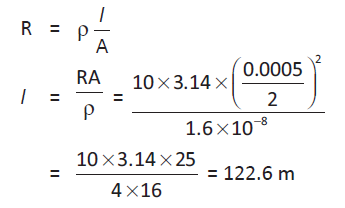∴ Length of the wire = 122.6 m

If the diameter of the wire is doubled, new diameter = 2 × 0.5 = 1 mm = 0.001 m.
Let new resistance be R′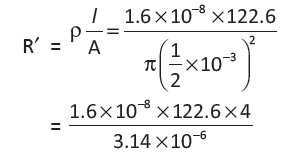Therefore, the length of the wire is 122.7 m and the new resistance is 2.5 Ω.
Q.7. The values of current I flowing in a given resistor for the corresponding values of potential difference V across the resistor are given below: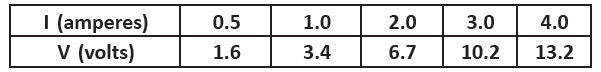Ans. The plot between voltage and current is called IV characteristic. The voltage is plotted on
x – axis and current is plotted on y-axis. The values of the current for different values of the voltage are shown in the given table.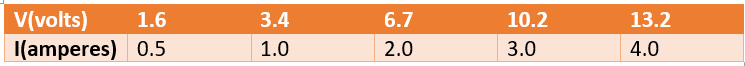The V–I characteristic of the given resistor is plotted in the following figure: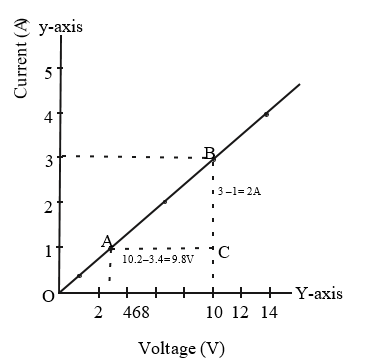The slope of the line gives the value of resistance (R) as,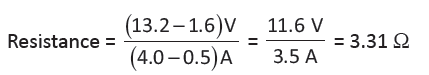Q.8. When a 12 V battery is connected across an unknown resistor, there is a current of 2.5 mA in the circuit. Find the value of the resistance of the resistor.
Ans. Resistance (R) of a resistor is given by Ohm’s law as, V = IR.
R = V/I
where, Potential difference, V = 12 V
Current in the circuit, I = 2.5 mA = $2.5 × 10^{-3} A$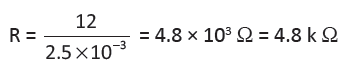Therefore, the resistance of the unknown resistor is 4.8 k Ω.
Q.9. A battery of 9 V is connected in series with resistors of 0.2 Ω, 0.3 Ω, 0.4 Ω, 0.5 Ω and 12 Ω, respectively. How much current would flow through the 12 Ω resistor?
Ans. There is no current division occurring in a series circuit. Current flow through the component is the same, given by Ohm’s law as
V = IR
I = V/R
where, R is the equivalent resistance of resistances 0.2 Ω, 0.3 Ω, 0.4 Ω, 0.5 Ω and 12 Ω. These are connected in series. Hence, the sum of the resistances will give the value of R.
R = 0.2 + 0.3 + 0.4 + 0.5 + 12 = 13.4 Ω
Potential difference, V = 9V
I = 9/13.4 = 0.671 A
Therefore, the current that would flow through the 12 Ω resistor is 0.671 A.
Q.10. How many 176 Ω resistors (in parallel) are required to carry 5 A on a 220 V line?
Ans. For x number of resistors of resistance 176 Ω, the equivalent resistance of the resistors connected in parallel is given by Ohm’s law as V = IR.
R = V/I
where, Supply voltage, V = 220 V
Current, I = 5 A
Equivalent resistance of the combination = R, given as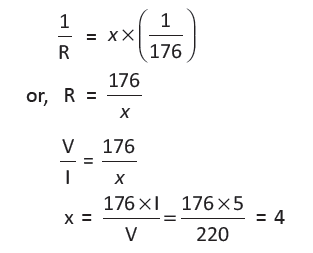Therefore, four resistors of 176 Ω are required to draw the given amount of current.
Q.11. Show how you would connect three resistors, each of resistance 6 Ω, so that the combination has a resistance of (i) 9 Ω, (ii) 4 Ω.
Ans. If we connect the resistors in series, then the equivalent resistance will be the sum of the resistors, i.e., 6 Ω + 6 Ω + 6 Ω = 18 Ω, which is not desired. If we connect the resistors in parallel, then the equivalent resistance will be 6/3 = 2 Ω is also not desired. Hence, we should either connect the two resistors in series or parallel.
(a) Two resistors in parallel.
Two 6 Ω resistors are connected in parallel. Their equivalent resistance will be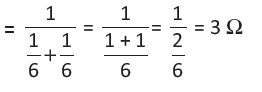The third 6 Ω resistor is in series with 3 Ω. Hence, the equivalent resistance of the circuit is 6 Ω + 3 Ω = 9 Ω.
(b) Two resistors in series.
Two 6 Ω resistors are in series. Their equivalent resistance will be the sum 6 + 6 = 12 Ω.
The third 6 Ω resistor is in parallel with 12 Ω. Hence, equivalent resistance will be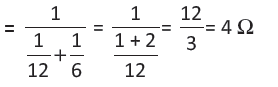Q.12. Several electric bulbs designed to be used on a 220 V electric supply line, are rated 10 W. How many lamps can be connected in parallel with each other across the two wires of 220 V line if the maximum allowable current is 5 A?
Ans. Resistance R1 of the bulb is given by the expression,
Supply voltage, V = 220 V
Maximum allowable current, I = 5 A
Rating of an electric bulb P = 10 watts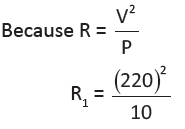According to Ohm’s law,
V = IR
Let R is the total resistance of the circuit for x number of electric bulbs
R = V/I
= 220/ 5 = 44Ω
Resistance of each electric bulb,$R_{1} = 4840 Ω$
$1/R = 1/R_{1} + 1/R_{1} + ……..$ upto x times.
$1/R = 1/R_{1}$ × x
$x = R_{1}/R$ = 4840/ 44
∴ Number of electric bulbs connected in parallel are 110.

Q.13. A hot plate of an electric oven connected to a 220 V line has two resistance coils A and B, each of 24 Ω resistances, which may be used separately, in series, or in parallel. What are the currents in the three cases?
Ans. Supply voltage, V= 220 V
Resistance of one coil, R= 24 Ω
(i) Coils are used separately
According to Ohm’s law ,
$V = I_{1}R_{1}$
where,$I_{1}$ is the current flowing through the coil
$I_{1} = V/ R_{1}= 220/24$
Therefore, 9.16 A current will flow through the coil when used separately.
(ii) Coils are connected in series
Total resistance, $R_{2} = 24 Ω + 24 Ω = 48 Ω$
According to Ohm’s law,$V = I_{2}R_{2}$
where,$I_{2}$ is the current flowing through the series circuit
$I_{2} = V/R_{2}$ = 220/48 = 4.58 A
Therefore, 4.58 A current will flow through the circuit when the coils are connected in series.
(iii) Coils are connected in parallel
Total resistance, $R_{3}$ is given as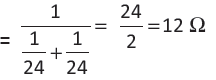= According to Ohm’s law,
$V = I_{3}R_{3}$
where,$I_{3}$ is the current flowing through the circuit $R_{3} = V/ R_{3}$
= 220/ 12 = 18.33 A
Therefore, 18.33 A current will flow through the circuit when coils are connected in parallel.
Q.14. Compare the power used in the 2 Ω resistor in each of the following circuits : (i) a 6 V battery in series with 1 Ω and 2 Ω resistors, and (ii) a 4 V battery in parallel with 12 Ω and 2 Ω resistors.
Ans. (i) Potential difference, V = 6 V
1 Ω and 2 Ω resistors are connected in series. Therefore, equivalent resistance of the circuit,
R = 1 + 2 = 3 Ω
According to Ohm’s law
V = IR
Where, I is the current through the circuit
I = 63 A = 2 A
This current will flow through each component of the circuit because there is no division of current in series circuits. Hence, current flowing through the 2 Ω resistor is 2A. Power is given by the expression,
$P_{1} = (I)^{2}R = (2)^{2} × 2 = 8 W$
(ii) Potential difference, V = 4 V
12 Ω and 2 Ω resistors are connected in parallel. The voltage across each component of a parallel circuit remains the same. Hence, the voltage across 2 Ω resistor will be 4 V.
Power consumed by 2 Ω resistor is given by
$P = V^{2}/R = 4^{2}/2 = 8 W$
Therefore, the power used by 2 Ω resistor is 8 W. Comparison between the power used in the cases $P_{1}/P_{2} = W_{1}/W_{2}$= 8/8 = 1

Q.15. Two lamps, one rated 100 W at 220 V, and the other 60 W at 220 V, are connected in parallel to electric mains supply. What current is drawn from the line if the supply voltage is 220 V?
Ans. Both the bulbs are connected in parallel. Therefore, potential difference across each of them will be 220 V, because no division of voltage occurs in a parallel circuit.
Current drawn by the bulb of rating 100 W is given by power = Voltage × Current
Current = Power/Voltage
= W/ V = 60/220
Hence, current drawn from the line = 100/ 220 + 60/ 220 = 0.727 A.
Q.16. Which uses more energy, a 250 W TV set in 1 hr, or a 1200 W toaster in 10 minutes?
Ans. Energy consumed by an electrical appliance is given by the expression, H = Pt
where, Power of the appliance = P
Time = t
Energy consumed by a TV set of power 250 W in 1 h = 250 × 3600
$= 9 × 10^{5} J$
Energy consumed by a toaster of power 1200 W in 10 minutes = 1200 × 600 = $7.2 × 10^{5} J$
Therefore, the energy consumed by a 250 W TV set in 1 h is more than the energy consumed by a toaster of power 1200 W in 10 minutes.
Q.17. An electric heater of resistance 8 Ω draws. 15 A from the service mains 2 hours. Calculate the rate at which heat is developed in the heater.
Ans. Rate of heat produced by a device is given by the expression for power as, P = $l^{2}R$
where, Resistance of the electric heater, R = 8 Ω
Current drawn, l = 15 A
$P = (15)^{2} × 8$= 1800 J/s
Therefore, heat is produced by the heater at the rate of 1800 J/s.
Q.18. Explain the following:
(a) Why is the tungsten used almost exclusively for filament of electric lamps?
(b) Why are the conductors of electric heating devices, such as bread-toaster and electric irons, made of an alloy rather than a pure metal?
(c) Why is the series arrangement not used for domestic circuits?
(d) How does the resistance of a wire vary with its area of cross-section?
(e) Why are copper and aluminium wires usually employed for electricity transmission?
Ans. (a) Tungsten has high melting point and emits light at high temperature.
(b) The conductors of electric heating devices such as bread toasters and electric irons are made of alloy because resistivity of an alloy is more than that of metals which produces large amount of heat.
(c) In series circuit voltage is divided. Each component of a series circuit receives a small voltage so the amount of current decreases and the device becomes hot and does not work properly. Hence, series arrangement is not used in domestic circuits.
(d) Resistance (R) of a wire is inversely proportional to its area of cross- section (A), i.e. when area of cross section increases the resistance decreases or vice versa.
(e) Copper and aluminium are good conductors of electricity also they have low resistivity. So they are usually used for electricity transmission.